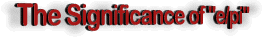By Richard C. Hoagland and Erol O. Torun Following these discoveries, the authors (this paper) began the current systematic inquiry into whether there was indeed a "message" at Cydonia: encoded geometrically in terms of specific placement of specific objects, by means of redundant mathematical ratios derived by dividing the observed angular relationships into one another. Over the last century or so, several prominent proposals have been made for encoding "CETI" messages by means of mathematical constants (Cocconi and Morrison, 1959; Sagan, 1973; Rubtsov and Ursal, 1984), and even physical geometric relationships on planetary surfaces (Gauss, et al., -- see Crowe, 1986). In particular, the authors were attempting to determine if e/pi = 0.865 [as opposed to the more fundamental ratio (sqrt 3)/2 = 0.866] was the ratio specifically intended at Cydonia. Others (notably Davies) had already raised key questions regarding this potential ambiguity. Other constants demonstrated at Cydonia by Hoagland and Torun being "sqrt 2," "3" and "sqrt 3" (1988), this confusion regarding which constant was "really" represented by the observed, redundant angle ratios, trig functions, and radian measure was considered an important question to resolve. Since "3" and "sqrt 3" are numbers essential to calculating "areas" and "volumes," Torun decided to explore their geometric implications first, following on Gauss (op cit). He began by investigating geometrical relationships among several fundamental "Platonic solids": the tetrahedron, cube, octahedron, icosohedron, and dodecahedron. In pursuing these explorations, Torun examined the mathematical properties of "circumscribed polyhedra" -- the Platonic solids embedded in a sphere. Almost immediately, he discovered something quite astonishing (to a non-specialist): the surface area of a tetrahedron (the "lowest order," simplest Platonic form), inscribed inside a "higher-order" form -- a sphere-- results in a surface ratio (sphere/tetrahedron) almost precisely equivalent to "e", the base of natural logarithms: e = 2.718282 surface of sphere ----------------------------------------------------   = 2.720699 surface of circumscribed tetrahedron Difference = 0.002417 The derivation of the above is as follows: (expressions are written in FORTRAN notation) Let A(t) = surface area of tetrahedron A(s) = surface area of circumscribing sphere R = radius of circumscribing sphere For a regular tetrahedron of edge a: A(t) = a**2 * sqrt(3) and R = a * sqrt(6)/4 For the circumscribing sphere: A(s) = 4*pi*R**2 = 4*pi * (a*sqrt(6)/4)**2 = (3/2)*pi*a**2 Area of sphere/area of circumscribed tetrahedron A(s)/A(t) = (3/2)*pi*a**2/(a**2 * sqrt(3)) = 3*pi/(2*sqrt (3)) A(s)/A(t) = 2.720699 - an approximation of e = 2.718282 When Torun substituted this "close approximation of e", termed e', in the equation most approximated at Cydonia: e/pi = 0.865 He discovered that: e'/pi = 2.720699/3.141593 = 0.866025 = (sqrt 3)/2 Or . . . precisely the observed "e/pi" ratio discovered at Cydonia!. The fact that e'/pi equals (sqrt 3)/2 can be demonstrated algebraically: Since e' was defined as 3*pi/(2*sqrt (3)), e'/pi = 3*pi/(2*sqrt (3)) / pi = 3/(2*sqrt (3)) = sqrt(3)/2 To place the above math in simple terms: The values of e/pi and (sqrt 3)/2 are precisely equal when e/pi is evaluated using the approximation of e that is generated by the geometry of a circumscribed tetrahedron. This simple fact completely resolves the ambiguity regarding which ratio -- e/pi or (sqrt 3)/2 -- was intended at Cydonia (see Fig. 4): Apparently, both were! Since the most redundantly observed Cydonia ratio is 0.866 and not 0.865 (the true ratio of the base of natural logarithms, divided by Pi -- to three significant-figures), it must now be clear, however, that the *primary* meaning of the "geometry of Cydonia" was in all likelihood intended to memorialize the (sphere)/(circumscribed tetrahedron) ratio [which is also (sqrt 3/2)], and not "e/pi". Further examples of "e/pi" at Cydonia -- appearing in connection with the ArcTan of 50.6 degrees (present at least twice in association with the Face) -- when examined by Hoagland, confirm that Torun's "circumscribed tetrahedral ratio" -- e' = 2.72069 -- and NOT the base of natural logarithms (e = 2.718282) provides a closer fit to the observed number. Thus strongly implying that "tetrahedral geometry" (and NOT the usual association of "e" with "growth equations") is the predominant meaning of "e/(sqrt 5)" and "(sqrt 5)/e" -- two other specific ratios found redundantly throughout the complex: e/(sqrt 5) = 1.215652 e'/(sqrt 5) = 1.216734 Cydonia ratio = 1.217 = ArcTan 50.6 degrees (The detailed implications of this association -- e' and (sqrt 5) -- will be examined in a subsequent paper.) These results, combined with other examples in the Complex (D&M Pyramid angles 60 degrees/ 69.4 degrees = 0.865 ) are what lead us to the conclusion that in fact *both* constants -- e and e' -- are deliberately encoded at Cydonia. In particular: D&M Pyramid apex = 40.868 deg N = ArcTan 0.865256 = e/pi But another feature on the D&M -- the wedge-shaped projection on the front -- defines the Pyramid's bilateral symmetry and orientation directly toward the Face. This feature also now seems to mark an equally important latitude: D&M "wedge" = 40.893 deg N = ArcTan 0.866025 = e'/pi = (sqrt 3)2 Torun identifies a conspicuous "knob," lying at the end of this wedge, as the "benchmark" designed to mark precisely the correct "e'/pi" latitude -- 40.893 degrees, approx. 1/40th degree North of the true apex of the Pyramid. The terminus of this wedge, together with the NW corner of the pyramid, are the only two points on the pyramid that, when connected, denote a line of latitude (see Fig. 5). Again, putting this in simple terms: The geometry of a circumscribed tetrahedron is not only suggested by the alignments in Cydonia, but also by the sitting latitude, size, shape, and orientation of the D&M Pyramid itself. This discovery only underscores the importance apparently attached to "circumscribed tetrahedral geometry" in the construction of Cydonia -- raising the important question: Why?